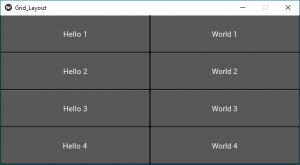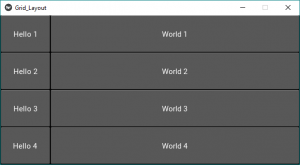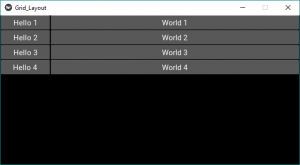# Python | Grid Layout in Kivy without .kv file

Kivy is a platform independent as it can be run on Android, IOS, linux and Windows etc. Kivy provides you the functionality to write the code for once and run it on different platforms. It is basically used to develop the Android application, but it Does not mean that it can not be used on Desktops applications.

### GridLayout:

• The widget must be placed in a specific column/row. Each child is automatically assigned a position determined by the layout configuration and the child’s index in the children list.
• Grid Layout must always contain any one of the below input constraints:
GridLayout.cols or GridLayout.rows. If you do not specify cols or rows, the Layout will throw an exception.
• The GridLayout arranges children in a matrix. It takes the available space and divides it into columns and rows, then adds widgets to the resulting “cells”.
• The row and coloums are just like the same as we observe in a matrix, here we can adjust the size of each grid.
• Initial the size is given by the col_default_width and row_default_height properties. We can force the default size by setting the col_force_default or row_force_default property. This will force the layout to ignore the width and size_hint properties of children and use the default size.

The first thing we need to do to use a GridLayout is to import it.

`from kivy.uix.gridlayout import GridLayout `

Basic Approach to create GridLayout:

```1) import kivy
2) import kivyApp
3) import button
4) import Gridlayout
5) Set minimum version(optional)
6) create App class:
- define build function
7) return Layout/widget/Class(according to requirement)
8) Run an instance of the class```

Implementation of the approach –

Code #1:
In the example below, all widgets will have an equal size. By default, the size_hint is (1, 1), so a Widget will take the full size of the parent:

 `# Sample Python application demonstrating   ` `# How to create GridLayout in Kivy  ` ` `  `# import kivy module  ` `import` `kivy  ` `   `  `# base Class of your App inherits from the App class.    ` `# app:always refers to the instance of your application    ` `from` `kivy.app ``import` `App  ` `   `  `# creates the button in kivy   ` `# if not imported shows the error   ` `from` `kivy.uix.button ``import` `Button ` ` `  `# The GridLayout arranges children in a matrix. ` `# It takes the available space and ` `# divides it into columns and rows, ` `# then adds widgets to the resulting “cells”. ` `from` `kivy.uix.gridlayout ``import` `GridLayout ` ` `  `# creating the App class  ` `class` `Grid_LayoutApp(App): ` ` `  `    ``# to build the application we have to ` `    ``# return a widget on the build() function.  ` `    ``def` `build(``self``): ` ` `  `        ``# adding GridLayouts in App ` `        ``# Defining number of coloumn ` `        ``# You can use row as well depends on need ` `        ``layout ``=` `GridLayout(cols ``=` `2``) ` ` `  `        ``# 1st row ` `        ``layout.add_widget(Button(text ``=``'Hello 1'``)) ` `        ``layout.add_widget(Button(text ``=``'World 1'``)) ` ` `  `        ``# 2nd row ` `        ``layout.add_widget(Button(text ``=``'Hello 2'``)) ` `        ``layout.add_widget(Button(text ``=``'World 2'``)) ` ` `  `        ``# 3rd row ` `        ``layout.add_widget(Button(text ``=``'Hello 3'``)) ` `        ``layout.add_widget(Button(text ``=``'World 3'``)) ` ` `  `        ``# 4th row ` `        ``layout.add_widget(Button(text ``=``'Hello 4'``)) ` `        ``layout.add_widget(Button(text ``=``'World 4'``)) ` ` `  `        ``# returning the layout ` `        ``return` `layout ` ` `  `# creating object of the App class ` `root ``=` `Grid_LayoutApp() ` `# run the App ` `root.run() `

Output:Now just change the class code in the above code with the code #2 and code#3 other than that all will be same as code#1 and run the code after changes you will get the below results.

Code #2:
Now, let’s fix the size of Hello buttons to 100px instead of using size_hint_x=1:

 `# creating the App class  ` `class` `Grid_LayoutApp(App): ` ` `  `    ``# to build the application we have to ` `    ``# return a widget on the build() function.  ` `    ``def` `build(``self``): ` ` `  `        ``# adding GridLayouts in App ` `        ``# Defining number of coloumn ` `        ``# You can use row as well depends on need ` `        ``layout ``=` `GridLayout(cols ``=` `2``) ` ` `  `        ``# 1st row ` `        ``layout.add_widget(Button(text ``=``'Hello 1'``, size_hint_x ``=` `None``, width ``=` `100``)) ` `        ``layout.add_widget(Button(text ``=``'World 1'``)) ` ` `  `        ``# 2nd row ` `        ``layout.add_widget(Button(text ``=``'Hello 2'``, size_hint_x ``=` `None``, width ``=` `100``)) ` `        ``layout.add_widget(Button(text ``=``'World 2'``)) ` ` `  `        ``# 3rd row ` `        ``layout.add_widget(Button(text ``=``'Hello 3'``, size_hint_x ``=` `None``, width ``=` `100``)) ` `        ``layout.add_widget(Button(text ``=``'World 3'``)) ` ` `  `        ``# 4th row ` `        ``layout.add_widget(Button(text ``=``'Hello 4'``, size_hint_x ``=` `None``, width ``=` `100``)) ` `        ``layout.add_widget(Button(text ``=``'World 4'``)) ` ` `  `        ``# returning the layout ` `        ``return` `layout `

Output:Code #3:
Now, let’s fix the row height to a specific size:

 `# creating the App class  ` `class` `Grid_LayoutApp(App): ` ` `  `    ``# to build the application we have to ` `    ``# return a widget on the build() function.  ` `    ``def` `build(``self``): ` ` `  `        ``# adding GridLayouts in App ` `        ``# Defining number of coloumn and size of the buttons i.e height ` `        ``layout ``=` `GridLayout(cols ``=` `2``, row_force_default ``=` `True``, ` `                            ``row_default_height ``=` `30``) ` ` `  `        ``# 1st row ` `        ``layout.add_widget(Button(text ``=``'Hello 1'``, size_hint_x ``=` `None``, width ``=` `100``)) ` `        ``layout.add_widget(Button(text ``=``'World 1'``)) ` ` `  `        ``# 2nd row ` `        ``layout.add_widget(Button(text ``=``'Hello 2'``, size_hint_x ``=` `None``, width ``=` `100``)) ` `        ``layout.add_widget(Button(text ``=``'World 2'``)) ` ` `  `        ``# 3rd row ` `        ``layout.add_widget(Button(text ``=``'Hello 3'``, size_hint_x ``=` `None``, width ``=` `100``)) ` `        ``layout.add_widget(Button(text ``=``'World 3'``)) ` ` `  `        ``# 4th row ` `        ``layout.add_widget(Button(text ``=``'Hello 4'``, size_hint_x ``=` `None``, width ``=` `100``)) ` `        ``layout.add_widget(Button(text ``=``'World 4'``)) ` ` `  `        ``# returning the layout ` `        ``return` `layout `

Output:My Personal Notes arrow_drop_upCheck out this Author's contributed articles.

If you like GeeksforGeeks and would like to contribute, you can also write an article using contribute.geeksforgeeks.org or mail your article to contribute@geeksforgeeks.org. See your article appearing on the GeeksforGeeks main page and help other Geeks.

Please Improve this article if you find anything incorrect by clicking on the "Improve Article" button below.

Article Tags :

Be the First to upvote.

Please write to us at contribute@geeksforgeeks.org to report any issue with the above content.